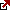# Present Value Continuous Compounding

Understanding Present Value Continuous Compounding Formula

Present value can be used with continuous compounding in order to find a solution to the equation. The formula is used to accurately calculate the current value of the future amount. This is useful and practical for a variety of different equations. There are a total of 3 different concepts to analyze in PV (present value). The first concept is known as time value. The second concept is known as the present value. The third concept is known as continuous composting. With diligent use of these 3 concepts, the present value formula with continuous compounding can be solved easily.

Present Value Continuous Compounding = C / e^rt, C is cash, r rate, t time.

Time value of money is an important concept that involves a few different aspects. The time value of money states that a specific amount of funds today is worth more than the same funds tomorrow. Time value of money is a very important concept in investments and other types of finances. There are many examples of time value for money. One main example of the time value of money would be a \$1,000 investment. The time value of money states that it is better to receive a \$1,000 investment today versus five years from now. This is one main example of how the time value of money works.

Present Value Continuous Compounding Calculator

The present value is the second concept stated above. Present value is similar to time value, in that time plays a large role in the outcome of a situation. Present value is commonly abbreviated “PV.” The present value involved calculating the present value of money at a later date. This may seem difficult, however with the right equation finding the PV is simple and easy.

Continuous compounding is the third concept stated above. Continuous compounding is used often in finance. Continuous compounding is very common for investments and other financial agreements. The compounding can take place on a weekly, monthly or annual basis. This is very important to understand as it reflects in the level of interest or increase. Cash flow is ultimately discounted by the rate factor for continuous compounding.

There are many practical equations that use present value. Using present value is a strategic method for solving the equation. Solving these types of equations is very important for accurately determining the final value of funds after time value, present value and continuous compounding.

The current amount needed to successfully achieve an \$1100 balance within two years is the variable being solved for. The answer to the equation would be 2 years which was the “T” variable. The “T” variable is very important as it represents time. Time is the main variable being solved as a result of its effect on the rest of the equation. The FV is also known as future value. The future value for this specific equation is \$1100. It is also important to note that 8% was the rate.

Latest posts by Fxigor (see all)

Fxigor

Igor has been a trader since 2007. Currently, Igor works for several prop trading companies. He is an expert in financial niche, long-term trading, and weekly technical levels. The primary field of Igor's research is the application of machine learning in algorithmic trading. Education: Computer Engineering and Ph.D. in machine learning. Igor regularly publishes trading-related videos on the Fxigor Youtube channel. To contact Igor write on: igor@forex.in.rs

Trade gold and silver. Visit the broker's page and start trading high liquidity spot metals - the most traded instruments in the world.